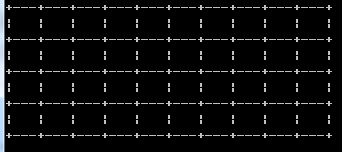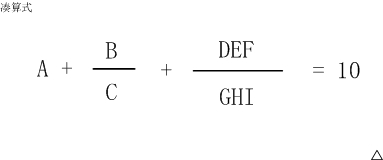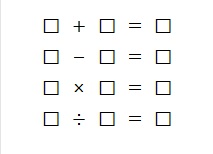# 第七届蓝桥杯试题及答案

X星球日报和我们地球的城市早报是一样的，

1125,1126,1727,1728

2*x-1=1125+1126+1727+1728解出x的值即可。这道题需要注意的是报纸的特殊情况第一面，第二面，最后一面，倒数第二面，是一页，不会的话买份报纸就知道了。

....

#include <bits/stdc++.h>

using namespace std;

int main()
{
int u=0;
int ans = 0;
for(int i=1;i<=100;i++)
{
u+=i;
ans+=u;
printf("%d\n",u);
}
printf("%d\n",ans);
return 0;
}

+---+---+---+---+---+---+---+---+---+---+
|   |   |   |   |   |   |   |   |   |   |
+---+---+---+---+---+---+---+---+---+---+
|   |   |   |   |   |   |   |   |   |   |
+---+---+---+---+---+---+---+---+---+---+
|   |   |   |   |   |   |   |   |   |   |
+---+---+---+---+---+---+---+---+---+---+
|   |   |   |   |   |   |   |   |   |   |
+---+---+---+---+---+---+---+---+---+---+

（如果显示有问题，可以参见【图1.jpg】）

#include <stdio.h>
//打印m列，n行的方格
void f(int m, int n)
{
int row;
int col;
for(row=0; row<n; row++){
for(col=0; col<m; col++) printf("+---");
printf("+\n");
for(col=0; col<m; col++) printf("|   ");
printf("|\n");
}
printf("+");
printf（“---+---+---+---+---+---+---+---+---+---+”）__;   //填空
printf("\n");
}

int main()
{
f(10,4);
return 0;
}#include <stdio.h>
void swap(int a[], int i, int j)
{
int t = a[i];
a[i] = a[j];
a[j] = t;
}
int partition(int a[], int p, int r)
{
int i = p;
int j = r + 1;
int x = a[p];
while(1){
while(i<r && a[++i]<x);
while(a[--j]>x);
if(i>=j) break;
swap(a,i,j);
}
___________ swap(a,p,j);___________;
return j;
}

void quicksort(int a[], int p, int r)
{
if(p<r){
int q = partition(a,p,r);
quicksort(a,p,q-1);
quicksort(a,q+1,r);
}
}

int main()
{
int i;
int a[] = {5,13,6,24,2,8,19,27,6,12,1,17};
int N = 12;
quicksort(a, 0, N-1);
for(i=0; i<N; i++) printf("%d ", a[i]);
printf("\n");
return 0;
}

B      DEF
A + --- + ------- = 10
C      GHI

（如果显示有问题，可以参见【图1.jpg】）

6+8/3+952/714 就是一种解法，
5+3/1+972/486 是另一种解法。#include <bits/stdc++.h>

using namespace std;

int main()
{
int sum=0;
for(float a=1;a<=9;a++)
{
for(float b=1;b<=9;b++)
{
for(float c=1;c<=9;c++)
{
for(float d=1;d<=9;d++)
{
for(float e=1;e<=9;e++)
{
for(float f=1;f<=9;f++)
{
for(float g=1;g<=9;g++)
{
for(float h=1;h<=9;h++)
{
for(float i=1;i<=9;i++)
{
if(a+b/c+(f+e*10+d*100)/(i+h*10+g*100)==10)
{
if((c!=0)&&(i+h*10+g*100!=0))
{
if((a!=b)&&(b!=c)&&(c!=d)&&(d!=e)&&(e!=f)&&(f!=g)&&(g!=h)&&(h!=i))
{
if((a!=c)&&(a!=d)&&(a!=e)&&(a!=f)&&(a!=g)&&(a!=h)&&(a!=i))
{
if((b!=d)&&(b!=e)&&(b!=f)&&(b!=g)&&(b!=h)&&(b!=i))
{
if((c!=e)&&(c!=f)&&(c!=g)&&(c!=h)&&(c!=i))
{
if((d!=f)&&(d!=g)&&(d!=h)&&(d!=i))
{
if((e!=g)&&(e!=h)&&(e!=i))
{
if((f!=h)&&(f!=i))
{
if(g!=i)
{
sum++;
printf("%.f %.f %.f %.f %.f %.f %.f %.f %.f\n",a,b,c,d,e,f,g,h,i);
}
}
}
}
}
}
}

}
}
}
}
}
}
}
}
}
}
}
}
printf("%d\n",sum);
return 0;
}

□ + □ = □
□ - □ = □
□ × □ = □
□ ÷ □ = □

(如果显示不出来，可以参见【图1.jpg】)

6  + 7 = 13
9  - 8 = 1
3  * 4 = 12
10 / 2 = 5

7  + 6 = 13
9  - 8 = 1
3  * 4 = 12
10 / 2 = 59,28,14,7,22,11,34,17,52,26,13,40,20,10,5,16,8,4,2,1

10

52

100

9232

CPU消耗  < 1000ms

#include <bits/stdc++.h>

using namespace std;

int main()
{
int n,max=0;
cin>>n;
for(int i=1;i<=n;i++)
{
int o=i;
if(i==1)
{
max=1;
}
else
{
while(i!=1)
{
if(i%2!=0)
{
i = i*3+1;
if(max<i)
max=i;
}
else
{
i=i/2;
if(max<i)
max=i;
}
}
i=o;
}
}
printf("%d\n",max);
return 0;
}

+---+---+---+
| A | * | * |
+---+---+---+
| B |   | * |
+---+---+---+

* A
**B

17

A B
***

12

CPU消耗  < 1000ms

X星球的考古学家发现了一批古代留下来的密码。

ABCBA

0

ABDCDCBABC

3

CPU消耗  < 3000ms05-282525

#### 蓝桥杯历年（省赛）试题汇总及试题详解©️2020 CSDN 皮肤主题: 编程工作室 设计师: CSDN官方博客点击重新获取扫码支付1.余额是钱包充值的虚拟货币，按照1:1的比例进行支付金额的抵扣。
2.余额无法直接购买下载，可以购买VIP、C币套餐、付费专栏及课程。余额充值